# Arithmetic Progressions Class 10 Extra Questions Maths Chapter 5 with Solutions Answers

Here we are providing Pair of Arithmetic Progressions Class 10 Extra Questions Maths Chapter 5 with Answers Solutions, Extra Questions for Class 10 Maths was designed by subject expert teachers.

## Extra Questions for Class 10 Maths Arithmetic Progressions with Answers Solutions

Extra Questions for Class 10 Maths Chapter 5 Arithmetic Progressions with Solutions Answers

### Arithmetic Progressions Class 10 Extra Questions Very Short Answer Type

Question 1.
Which of the following can be the nth term of an AP?
4n + 3, 3n2 + 5, n2 + 1 give reason.
Solution:
4n + 3 because nth term of an AP can only be a linear relation in n as an = a + (n – 1)d.

Question 2.
Is 144 a term of the AP: 3, 7, 11, …? Justify your answer.
Solution:
No, because here a = 3 an odd number and d = 4 which is even. so, sum of odd and even must be odd whereas 144 is an even number.

Question 3.
The first term of an AP is p and its common difference is q. Find its 10th term.
Solution:
210 = a + 9d = p + 99.

Question 4.
For what value of k: 2k, k + 10 and 3k + 2 are in AP?
Solution:
Given numbers are in AP
∴ (k + 10) – 2k = (3k + 2) – (k + 10)
⇒ -k + 10 = 2k – 8 or 3k = 18 or k = 6.

Question 5.
If an = 5 – 11n, find the common difference.
Solution:
We have an = 5 – 11n
Let d be the common difference
d = an+1 – an
= 5 – 11(n + 1) – (5 – 11n)
= 5 – 11n – 11 -5 + 11n = -11

Question 6.
If nth term of an AP is $$\frac{3+n}{4}$$ find its 8th term.
Solution: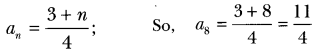Question 7.
For what value of p are 2p + 1, 13, 5p – 3, three consecutive terms of AP?
Solution:
since 20 + 1, 13, 5p – 3 are in AP.
∴ second term – First term = Third term – second term
⇒ 13 – (2p + 1) = 5p – 3 – 13
⇒ 13 – 2p – 1 = 5p – 16
⇒ 12 – 2p = 5p – 16
⇒ -7p = – 28
⇒ p = 4

Question 8.
In an AP, if d = -4, n = 7, a, = 4 then find a.
Solution:
We know, an = a + (n – 1)d
Putting the values given, we get
⇒ 4 = a + (7 – 1)(-4) or a = 4 + 24
⇒ a = 28

Question 9.
Find the 25th term of the AP: -5, $$\frac{-5}{2}$$ , 0, $$\frac{-5}{2}$$ ………
Solution:
Here, a = -5, b = –$$\frac{5}{2}$$ – (-5) = $$\frac{5}{2}$$
We know,
a25 = a + (25 – 1 )d
= (-5) + 24($$\frac{5}{2}$$) = -5 + 60 = 55

Question 10.
Find the common difference of an AP in which a18 – a14 = 32.
Solution:
Given, a18 – a14 = 32
⇒ (a + 17d) – (a + 13d) = 32
⇒ 17d – 13d = 32 or d = $$\frac{32}{4}$$

Question 11.
If 7 times the 7th term of an AP is equal to 11 times its 11th term, then find its 18th term.
Solution:
Given, 7a7= 11a11
⇒ 7(a + 6d) = 11(a + 100) or 7a + 42d = 11a + 110d
⇒ 4a + 68d = 0 or a + 17d = 0
Now, a18 = a + 17d = 0

Question 12.
In an AP, if a = 1, an = 20, and sn = 399, then find n.
Solution:
Given, An = 20
= 1 + (n – 1)d = 20
⇒ (n – 1) d = 19
sn = $$\frac{n}{2}$$ {2a + (n – 1)d}
⇒ 399 = $$\frac{n}{2}$${2 × 1 + 19}
⇒ $$\frac{399 \times 2}{21}$$ = n
⇒ n = 38

Question 13.
Find the 9th term from the end (towards the first term) of the AP 5, 9, 13, …, 185.
Solution:
l = 185, d = 4
l9 = l – (n – 1) d
= 185 – 8 × 4 = 153

### Arithmetic Progressions Class 10 Extra Questions Short Answer Type 1

Question 1.
In which of the following situations, does the list of numbers involved to make an AP? If yes, give a reason.
(i) The cost of digging a well after every meter of digging, when it costs 150 for the first meter and rises by 50 for each subsequent meter.
(ii) The amount of money in the account every year, when 10,000 is deposited at simple interest at 8% per annum.
Solution:
(i) The numbers involved are 150, 200, 250, 300, …
Here 200 -150 = 250 – 200 = 300 – 250 and so on
∴ It forms an AP with a = 150, d = 50

(ii) The numbers involved are 10,800, 11,600, 12,400, …
which forms an AP with a = 10,800 and d = 800.

Question 2.
Find the 20th term from the last term of the AP: 3, 8, 13, …, 253.
Solution:
We have, last term = 1 = 253
And, common difference d = 2nd term – 1st term = 8 – 3 = 5
Therefore, 20th term from end = 1 -(20 – 1) × d = 253 – 19 × 5 = 253 – 95 = 158.

Question 3.
If the sum of the first p terms of an AP is ap2 + bp, find its common difference.
Solution:
ap = sp – sp-1 = (ap2 + bp) -[a(p – 1)2 + b(p – 1)]
= ap2 + bp – (ap2 + a – 2ap + bp – b)
= ap2 + bp – ap2 – a + 2ap – bp + b = 2ap + b-a .
= a1 = 2a + b – a = a + b and a2 = 4a + b – a = 3a + b
⇒ d = a2 – a1 = (3a + b) – (a + b) = 2a

Question 4.
The first and the last terms of an AP are 5 and 45 respectively. If the sum of all its terms is 400, find its common difference.
Solution:
Let the first term be ‘a’ and common difference be ‘d’.
Given, a = 5, Tn = 45, sn = 400 .
Tn = a + (m – 1)d
⇒ 45 = 5 + (m – 1)d
⇒ (n – 1) d = 40 ………(i)
sn = $$\frac{n}{2}$$ (a + Tn)
⇒ 400 = $$\frac{n}{2}$$ (5 + 45)
⇒ n = 2 × 8 = 16 substituting the value of n in (i)
⇒ (16 – 1)d = 40
⇒ d = $$\frac{40}{15}$$ = $$\frac{8}{3}$$

Question 5.
Find the number of natural numbers between 101 and 999 which are divisible by both 2 and 5.
Solution:
Natural numbers between 101 and 999 divisible by both 2 and 5 are 110, 120, … 990.
so, a1 = 110, d = 10, an = 990
We know, an = a1 + (n – 1)d
990 = 110 + (n – 1) 10
(n – 1) = $$\frac{990-110}{10}$$
⇒ n = 88 + 1 = 89

Question 6.
Find how many integers between 200 and 500 are divisible by 8.
Solution:
AP formed is 208, 216, 224, …, 496
Here, an = 496, a = 208, d = 8
an = a + (n – 1) d
⇒ 208 + (n – 1) x 8 = 496
⇒ 8 (n – 1) = 288
⇒ n – 1 = 36
⇒ n = 37

Question 7.
The sum of the first n terms of an AP is 3n2 + 6n. Find the nth term of this
Solution:
Given: sn = 3n2 +6n
sn-1 = 3(n – 1)2 + 6(n – 1)
⇒ 3(n2 + 1 – 2n) + 6n – 6
⇒ 3m2 + 3 – 6n + 6n – 6 = 3n2 – 3
The nth term will be an
sn = sn-1 + an
an = sn – sn-1
⇒ 3n2 + 6n – 3n2 + 3
⇒ 6n + 3

Question 8.
How many terms of the AP 18, 16, 14, …. be taken so that their sum is zero?
Solution:
Here, a = 18, d = -2, sn = 0
Therefore, $$\frac{n}{2}$$ [36 + (n – 1) (- 2)] = 0
⇒ n(36 – 2n + 2) = 0
⇒ n(38 – 2n) = 0
⇒ n = 19

Question 9.
The 4th term of an AP is zero. Prove that the 25th term of the AP is three times its 11th term.
Solution:
∵ a4 = 0 (Given)
⇒ a + 3d = 0
⇒ a = -3d
a25 = a + 24d = – 3d + 24d = 21d
3a11 = 3(a + 100) = 3(70) = 21d
∴ a25 = 3011
Hence proved.

Question 10.
If the ratio of sum of the first m and n terms of an AP is m2 : n2, show that the ratio of its mth and nth terms is (2m – 1) : (2n – 1).
Solution: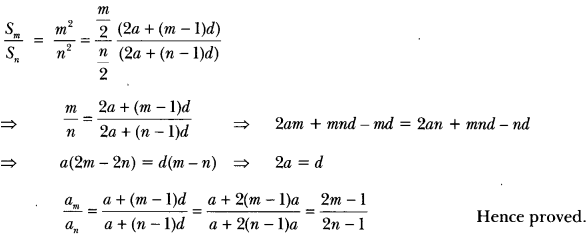Question 11.
What is the common difference of an AP in which a21 – a7 = 84?
Solution:
Given: a21 – a7 = 84
⇒ (a + 20d) – (a + 6d) = 84
⇒ 14d = 84
⇒ d = 6

Question 12.
For what value of n, are the nth terms of two APs 63, 65, 67,… and 3, 10, 17,… equal?
Solution:
Let nth terms for two given series be an and a’n
According to questions
an = a’n
⇒ a + (n – 1)d = a + (n – 1)d’
⇒ 63 + (n – 1)2 = 3 + (n – 1)7
⇒ 5n = 65
⇒ n = 13.

### Arithmetic Progressions Class 10 Extra Questions Short Answer Type 2

Question 1.
Which term of the AP: 3, 8, 13, 18, … , is 78?
Solution:
Let an be the required term and we have given AP
3, 8, 13, 18, …..
Here, a = 3, d = 8 – 3 = 5 and an = 78
Now, an = a + (n – 1)d
⇒ 78 = 3 + (n – 1) 5
⇒ 78 – 3 = (n – 1) × 5
⇒ 75 = (n – 1) × 5
⇒ $$\frac{75}{5}$$ = n – 1
⇒ 15 = n – 1
⇒ n = 15 + 1 = 16
Hence, 16th term of given AP is 78.

Question 2.
Find the 31st term of an AP whose 11th term is 38 and the 16th term is 73.
Solution:
Let the first term be a and common difference be d.
Now, we have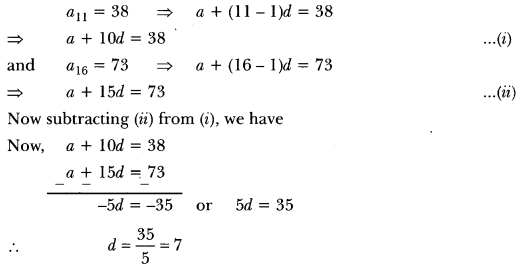Putting the value of d in equation (i), we have
a + 10 × 7 = 38
⇒ a + 70 = 38
⇒ a = 38 – 70
⇒ a = – 32
We have, a = -32 and d = 7
Therefore, a31 = a + (31 – 1)d
⇒ a31 = a + 30d
⇒ (-32) + 30 × 7
⇒ – 32 + 210
= a31 = 178

Question 3.
An AP consists of 50 terms of which 3rd term is 12 and the last term is 106. Find the 29th term.
Solution:
Let a be the first term and d be the common difference.
since, given AP consists of 50 terms, so n = 50
a3 = 12
⇒ a + 2d = 12 …(i)
Also, a50 = 106
⇒ a + 490 = 106 … (ii)
subtracting (i) from (ii), we have
47d = 94
⇒ d = $$\frac{94}{47}$$ = 2
Putting the value of d in equation (i), we have
a + 2 × 2 = 12
⇒ a = 12 – 4 = 8
Here, a = 8, d = 2
∴ 29th term is given by
a29 = a + (29 – 1)d = 8 + 28 × 2
⇒ a29 = 8 + 56
⇒ a29 = 64

Question 4.
If the 8th term of an AP is 31 and the 15th term is 16 more than the 11th term, find the AP.
Solution:
Let a be the first term and d be the common difference of the AP.
We have, a8 = 31 and a15 = 16 + a11
⇒ a + 7d = 31 and a + 14d = 16 + a + 10d
⇒ a + 7d = 31 and 4d = 16
⇒ a + 7d = 31 and d = 4
⇒ a + 7 × 4 = 31
⇒ a + 28 = 31
⇒ a= 3
Hence, the AP is a, a + d, a + 2d, a + 3d…..
i.e., 3, 7, 11, 15, 19, …

Question 5.
Which term of the arithmetic progression 5, 15, 25, …. will be 130 more than its 31st term?
Solution:
We have, a = 5 and d = 10
∴ a31 = a + 30d = 5 + 30 × 10 = 305
Let nth term of the given AP be 130 more than its 31st term. Then,
an = 130 + a31
∴ a + (n – 1)d = 130 + 305
⇒ 5 + 10(n – 1) = 435
⇒ 10(n – 1) = 430
⇒ n – 1 = 43
⇒ n = 44
Hence, 44th term of the given AP is 130 more than its 31st term.

Question 6.
Which term of the progression $$20,19 \frac{1}{4}, 18 \frac{1}{2}, 17 \frac{3}{4}$$, is the first negative term?
Solution: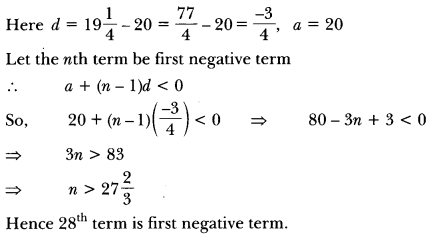Question 7.
Find the sum given below:
7 + 10 $$\frac{1}{2}$$ + 14 +…+ 84
Solution:
Let a be the first term, d be the common difference and an be the last term of given AP.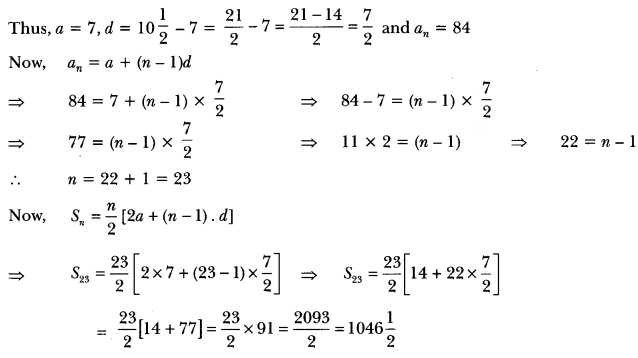Question 8.
In an AP: given l = 28, s = 144, and there are total 9 terms. Find a.
Solution:
We have, l = 28, s = 144 and n = 9
Now, l = an = 28
28 = a + (n – 1) d 28 = a + (9 – 1)d
⇒ 28 = a + 8d ……(i)
and S = 144
⇒ 141 = $$\frac{1}{2}$$ [2a + (n – 1)d]
⇒ 144 = $$\frac{9}{2}$$ [12a +(9 – 1) d]
$$\frac{144 \times 2}{9}$$ = 2a + 8d
⇒ 32 = 2a + 8d
⇒ 16 = a + 4d … (ii)
Now, subtracting equation (ii) from (i), we get
4d = 12 or d = 3
Putting the value of d in equation (i), we have
a + 8 × 3 = 28
⇒ a + 24 = 28
⇒ a = 28 – 24
∴ a = 4.

Question 9.
How many terms of the AP: 9, 17, 25, … must be taken to give a sum of 636?
Solution:
Let sum of n terms be 636.
sn = 636, a = 9, d = 17 – 9 = 8
⇒ $$\frac{n}{2}$$[2a + (n – 1) d] = 636
⇒ $$\frac{n}{2}$$[2 x 9 + (n – 1) × 8] = 636
⇒ $$\frac{n}{2}$$ × 2[9+ (n – 1) 4] = 636
⇒ n[9 + 4n – 4] = 636
⇒ n[5 + 4n] = 636
⇒ 5n + 4n2 = 636
⇒ 4n2 + 5n – 636 = 0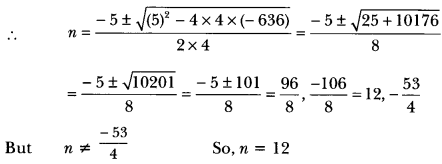Thus, the sum of 12 terms of given AP is 636.

Question 10.
How many terms of the series 54, 51, 48 ……. be taken so that, their sum is 513? Explain the
Solution:
Clearly, the given sequence is an AP with first term a = 54 and common difference d = -3. Let
the sum of n terms be 513. Then,
sn = 513
⇒ $$\frac{n}{2}$$ {2a + (n – 1) d} = 513
⇒ $$\frac{n}{2}$$ (108 + (n – 1) – 3) = 513
⇒ n[108 – 3n + 3) = 1026
⇒ -3n2 + 111n = 1026
⇒ n2 – 37n + 342 = 0
⇒ (n – 18) (n – 19) = 0
⇒ n = 18 or 19
Here, the common difference is negative. so, 19th term is given by
a19 = 54 + (19 – 1) × – 3 = 0
Thus, the sum of 18 terms as well as that of 19 terms is 513.

Question 11.
The first term, common difference and last term of an AP are 12, 6 and 252 respectively. Find the sum of all terms of this AP.
Solution:
We have, a = 12, d = 6 and l = 252
Now, l = 252
⇒ an = 252
= l = a + (n – 1)d
⇒ 252 = 12 + (n – 1) × 6
⇒ 240 = (n – 1) × 6
⇒ n – 1 = 40 or n = 41
Thus, Sn = $$\frac{n}{2}$$(a + l)
⇒ S41 = $$\frac{41}{2}$$(12 + 252) = $$\frac{41}{2}$$ (264) = 41 × 132 = 5412

Question 12.
If the sum of first 7 terms of an AP is 49 and that of 17 terms is 289, find the sum of first n terms.
Solution:
We have, S7 = 49
⇒ 49 = $$\frac{7}{2}$$[2a + (7 – 1) × d]
⇒ 49 × $$\frac{2}{7}$$ = 2a + 6d
⇒ 14 = 2a + 6d
⇒ a + 3d = 7
and S17 = 289
⇒ 289 = $$\frac{17}{2}$$ [2a + (17 – 1)d]
⇒ 2a + 16d = $$\frac{289 \times 2}{17}$$ = 34
⇒ a + 8d = 17
Now, subtracting equation (i) from (ii), we have
5d = 10 ⇒ d = 2
Putting the value of d in equation (i), we have
a + 3 × 2 = 7
⇒ a = 7 – 6 = 1
Here, a = 1 and d = 2
Now, sn = $$\frac{n}{2}$$ [2a + (n – 1) d]
= $$\frac{n}{2}$$ [2 × 1 + (n – 1) × 2]
= $$\frac{n}{2}$$[2 + 2n – 2]
= $$\frac{n}{2}$$ × 2n = n2

Question 13.
The first term of an AP is 5, the last term is 45 and the sum is 400. Find the number of terms and the common difference.
Solution: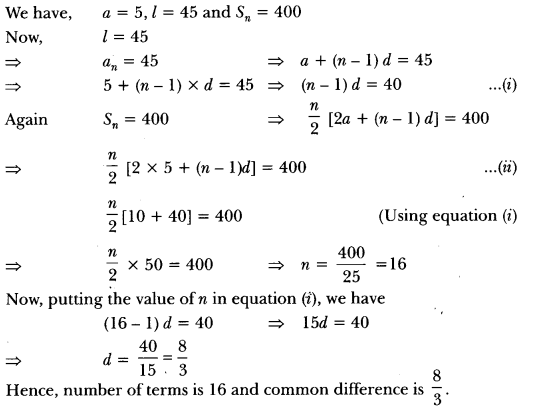Question 14.
If the seventh term of an AP is a and its ninth term is, find its 63rd term.
Solution: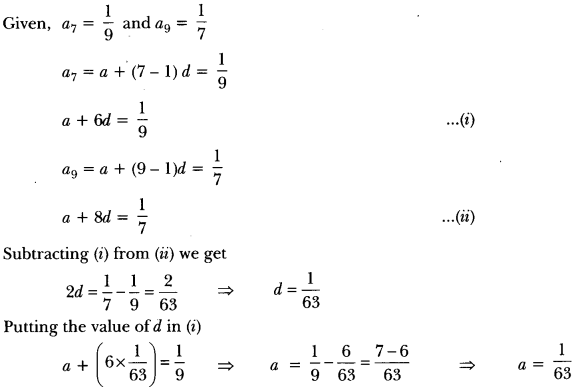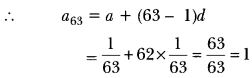Question 15.
The sum of the 5th and the 9th terms of an AP is 30. If its 25th term is three times its 8th term, find the AP.
Solution:
a5 + a9 = 30
⇒ (a + 4d) + (a + 8d) = 30 = 2a + 12d = 30
⇒ a + 6d = 15
a = 15 – 6d …(i)
a25 = 3a8
⇒ a + 24d = 3(a + 7d)
á + 24d = 3a + 21d
⇒ 2a = 3d
Putting the value of a form (i), we have
2(15 – 6d) = 3d
⇒ 30 – 12d = 3d
⇒ 15d = 30
⇒ d = 2
So, a = 15 – 6 × 2 = 15 – 12 [From equation (i)]
⇒ a = 3
The AP will be 3, 5, 7, 9….

Question 16.
The sum of the first 7 terms of an AP is 63 and the sum of its next 7 terms is 161. Find the 28th term of this AP.
Solution:
sum of first seven terms,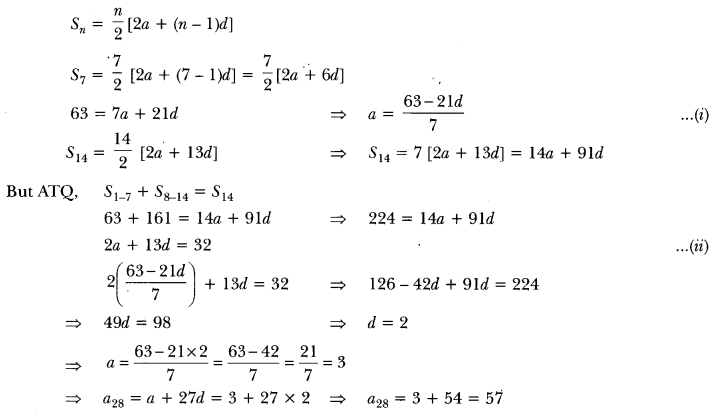Question 17.
If the ratio of the sum of first n terms of two AP’s is (7n + 1): (4n + 27), find the ratio of their mth terms.
Solution: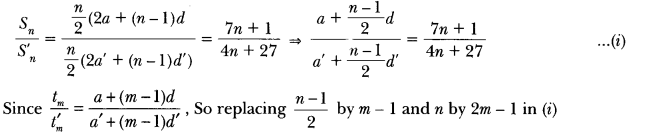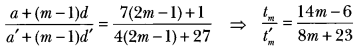Question 18.
Find the sum of the following series :
5 + (-41) + 9 + (-39) + 13 + (-37) + 17 + … + (-5) + 81 + (-3)
Solution:
The series can be rewritten as,
(5 + 9 + 13 + … +81) + (41 + (-39) + (-37) + … + (-5) + (-3))
For the series 5 + 9 + 13 + … 81
a = 5, d = 4 and an = 81
nth term = a + (n – 1)d = an
⇒ 5+ (n – 1)4 = 81
⇒ 4n = 80
⇒ n =
20 sum of 20 terms for this series.
sn = $$\frac{20}{2}$$ (5 + 81) = 860 …(i)
[∵ sn = $$\frac{n}{2}$$ (a + an)]
For the series (41) + (-39) + (-37)… + (-5) + (-3)
a = 41, d = 2 and and = -3
nth term = a + (n – 1)d = an.
⇒ -41 + (n – 1)2 = -3
⇒ 2n = 40
⇒ n = 20
sum of 20 terms for this series
sn = $$\frac{20}{2}$$ (-41 – 3) = – 440 …(ii)
By adding (i) and (ii), we get
sum of series = 860 – 440
= 420

Question 19.
Find the sum of all two digit natural numbers which are divisible by 4.
Solution:
Here a = 12, d = 4, an = 96
The formula is an = a + (n – 1)d
Therefore 96 = 12 + (n – 1) × 4
⇒ 96 = 8 + 4n
⇒ n = $$\frac{88}{4}$$
⇒ n = 22
Apply the formula for sum,
sn = $$\frac{n}{2}$$ [2a + (n – 1)d]
Hence, s22 = 11/24 + 21 × 4] = 11[24 + 84]
= 11 × 108 = 1188.

### Arithmetic Progressions Class 10 Extra Questions Long Answer Type

Question 1.
The sum of the 4th and 8th term of an AP is 24 and the sum of the 6th and 10th term is 44. Find the first three terms of the AP.
Solution:
We have, a4 + a8 = 24
⇒ a + (4 – 1)d + a + (8 – 1) d = 24
⇒ 2a + 3d + 7d = 24
⇒ 2a + 10d = 24
⇒ 2(a + 5d) = 24
∴ a + 5d = 12
and, a6 + a10 = 44
⇒ a + (6 – 1)d + a + (10 – 1) d = 44
⇒ 2a + 5d + 9d = 44
⇒ 2a + 14d = 44
⇒ a + 7d = 22
subtracting (i) from (ii), we have
2d = 10
∴d = $$\frac{10}{2}$$ = 5
Putting the value of d in equation (i), we have
a + 5 × 5 = 12
⇒ a = 12 – 25 = -13
Here, a = -13, d = 5
Hence, first three terms are
-13, -13, + 5, -13 + 2 × 5 i.e., -13, -8, -3

Question 2.
The sum of the first n terms of an AP is given by sn = 3n2 – 4n. Determine the AP and the 12th term.
Solution:
We have, sn = 3n2 – 4n …(i)
Replacing n by (n – 1), we get
sn-1 = 3(n – 1)2 – 4(n – 1) ….(ii)
We know, .
an = sn – sn-1 = {3n2 – 4n} – {3(n – 1)2 – 4(n – 1)}.
= {3n2 – 4n} – {3n2 + 3 – 6n – 4n + 4}
= 3n2 – 4n – 3n2 – 3 + 6n + 4n – 4 = 6n – 7
so, nth term an = 6n – 7
To get the AP, substituting n = 1, 2, 3… respectively in (iii), we get
a1 = 6 × 1-7 = -1,
a2 = 6 × 2 – 7 = 5
a3 = 6 × 3 – 7 = 11,…
Hence, AP is – 1,5, 1:1, …
Also, to get 12th term, substituting n = 12 in (iii), we get
a12 = 6 × 12 – 7 = 72 – 7 = 65

Question 3.
Divide 56 into four parts which are in AP such that the ratio of product of extremes to the product of means is 5 : 6.
Solution:
Let the four parts be a – 3d, a-d, a + d, a + 3d.
Given, (a – 3d) + (a – d) + (a + d) + (a + 3d) = 56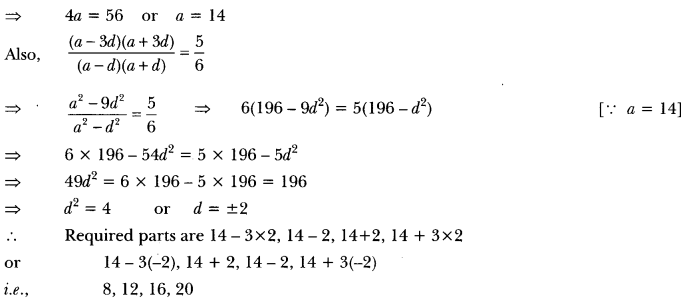Question 4.
In an AP of 50 terms, the sum of first 10 terms is 210 and the sum of its last 15 terms is 2565. Find the AP.
Solution:
Let ‘a’ be the first term and ‘d be the common difference.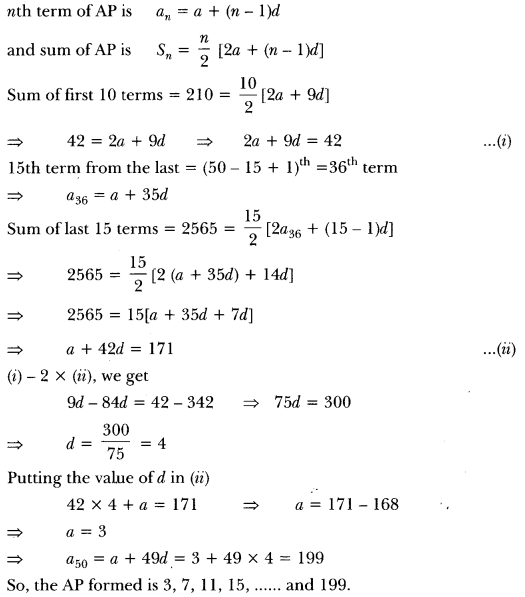Question 5.
If s, denotes the sum of the first n terms of an AP, prove that s30 = 3 (s20 – s10).
Solution: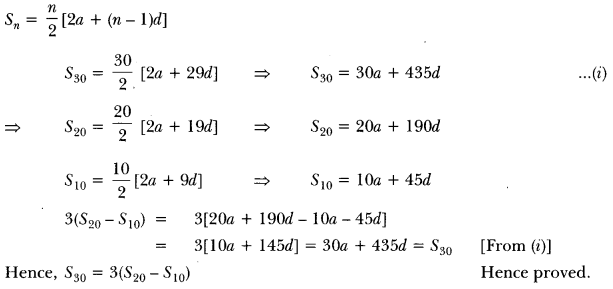Question 6.
A thief runs with a uniform speed of 100 m/minute. After one minute a policeman runs after the thief to catch him. He goes with a speed of 100 m/minute in the first minute and increases his speed by 10 m/minute every succeeding minute. After how many minutes the policeman will catch the thief?
Solution:
Let total time be n minutes
Total distance covered by thief = 100 n metres
Total distance covered by policeman = 100 + 110 + 120 + … + (n – 1) terms
∴ 100m = $$\frac{n-1}{2}$$ [100(2) + (n – 2)10]
⇒ 200n = (n – 1)(180 + 10n)
⇒ 102– 30n – 180 = 0
⇒ n2 – 3n – 18 = 0
⇒ (n-6) (n + 3) = 0
⇒ n = 6
Policeman took (n – 1) = (6 – 1) = 5 minutes to catch the thief.

Question 7.
The houses in a row are numbered consecutively from 1 to 49. show that there exists a value of X such that sum of numbers of houses preceeding the house numbered X is equal to sum of the numbers of houses following X. Find value of X.
Solution:
The numbers of houses are 1, 2, 3, 4……….49.
The numbers of the houses are in AP, where a = 1 and d = 1
sum of n terms of an AP = $$\frac{n}{2}$$[2a + (n – 1)d]
Let Xth number house be the required house.
sum of number of houses preceding Xth house is equal to sx-1 i.e.,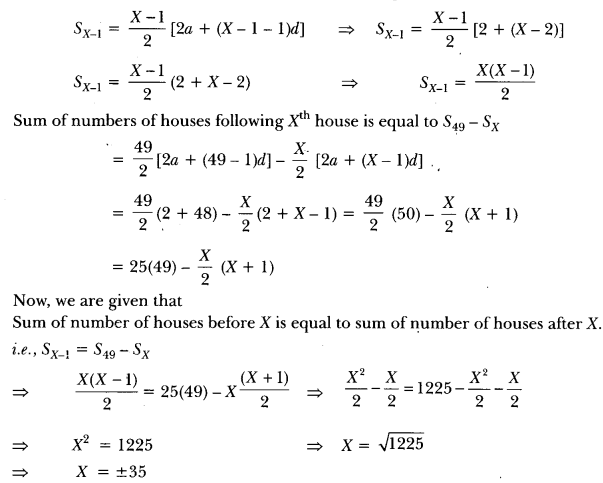since number of houses is positive integer,
∴ X = 35

Question 8.
If the ratio of the 11th term of an AP to its 18th term is 2:3, find the ratio of the sum of the first five terms to the sum of its first 10 terms.
Solution: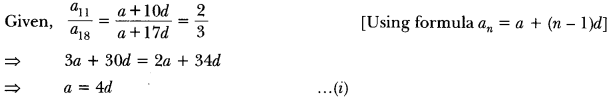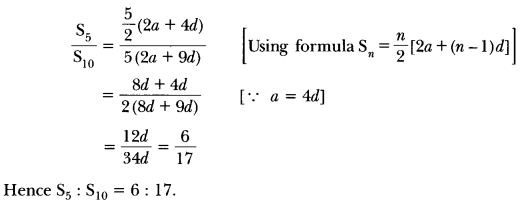### Arithmetic Progressions Class 10 Extra Questions HOTS

Question 1.
Find the sum of the first 15 multiples of 8.
Solution:
The first 15 multiples of 8 are
8, 16, 24, … 120
Clearly, these numbers are in AP with first term a = 8 and common difference, d = 16 – 8 = 8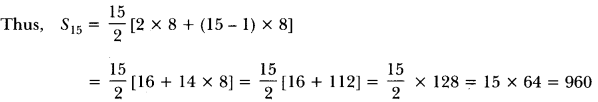Question 2.
Find the sum of all two digit natural numbers which when divided by 3 yield 1 as remainder.
Solution:
Two digit natural numbers which when divided by 3 yield 1 as remainder are:
10, 13, 16, 19, …, 97, which forms an AP.
with a = 10, d = 3, an = 97
an = 97 = a + (n – 1) d = 97
or 10 + (n – 1)3 = 97
⇒ (n – 1) = $$\frac{87}{3}$$ = 29
⇒ n = 30
Now, s30 = [2 × 10 + 29 × 3) = 15(20 + 87) = 15 × 107 = 1605

Question 3.
A sum of ₹700 is to be used to give seven cash prizes to students of a school for their overall academic performance. If each prize is ₹20 less than its preceding prize, find the value of each of the prizes.
Solution:
Let the prizes be a + 60, a + 40, a + 20, a, a – 20, a – 40, a – 60
Therefore, the sum of prizes is
a + 60 + a + 40 + a + 20 + a ta – 20 + a – 40 +a – 60 = 700
= 7a = 700
⇒ a = $$\frac{700}{7}$$ = 100
Thus, the value of seven prizes are
100 + 60, 100 + 40, 100 + 20, 100, 100 – 20, 100 – 40, 100 – 60
i.e., ₹160, ₹140, ₹120, ₹100, ₹80, ₹60, ₹340

Question 4.
If the mth term of an AP is $$\frac{1}{n}$$ and nth term is $$\frac{1}{m}$$, then show that its (mn)th term is 1.
Solution:
Let a and d be the first term and common difference respectively of the given AP. Then
am = a + (m – 1) d
⇒ a + (m – 1)d = $$\frac{1}{n}$$ …… (i)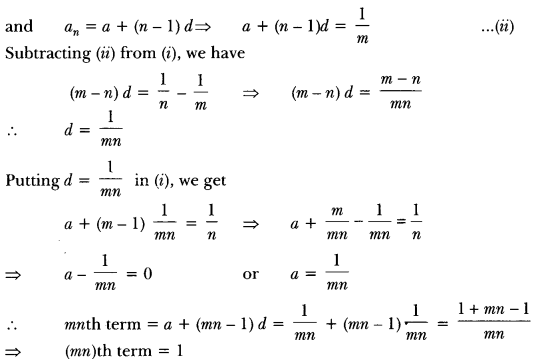Question 5.
If the sum of m terms of an AP is the same as the sum of its n terms, show that the sum of its (m + n) terms is zero.
Solution:
Let a be the first term and d be the common difference of the given AP.
Then, sm = sn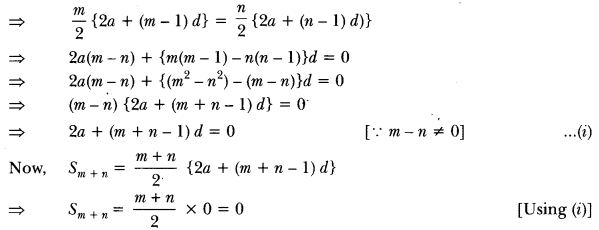Question 6.
The ratio of the sums of m and n terms of an AP is m2 : n2. show that the ratio of the mth and nth terms is (2m – 1): (2n – 1).
Solution:
Let a be the first term and d the common difference of the given AP. Then, the sums of m and n terms are given by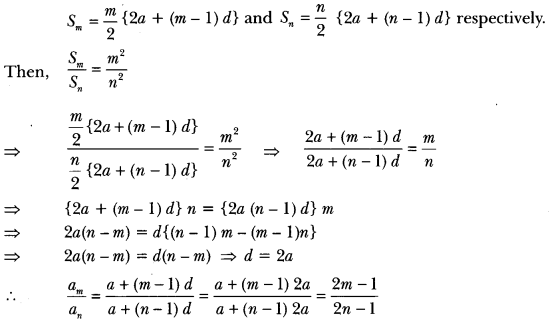Question 7.
The sum of n, 2n, 3n terms of an AP are s1, s2, and s3 respectively. Prove that s3 = 3(s2 – s1).
Solution:
Let a be the first term and d be the common difference of the AP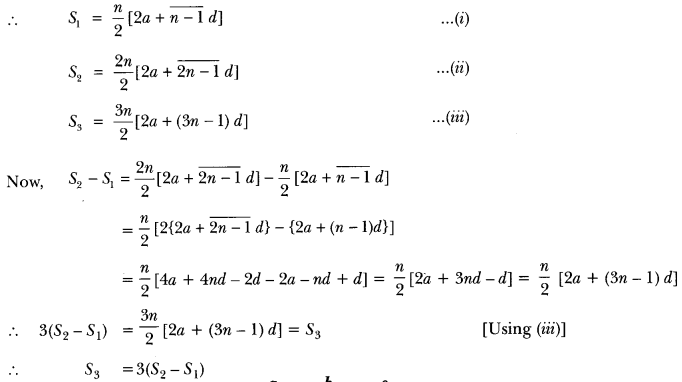Question 8.
If a2, b2, c2, are in AP, prove that $$\frac{a}{b+c}, \frac{b}{c+a}, \frac{c}{a+b}$$ are in AP.
Solution:
since a2, b2, c2, are in AP …(i)